# Nios Solutions for Maths## Terminal Exercise Limits and continuity Solutions NIOS maths 311 book 2

NIOS Mathematics 311 book 2 senior secondary Module – VIII; Calculus Chapter 25 Limits and Continuity Terminal Exercise solutions are given. You should study the textbook lesson Limits and Continuity very well. You should practice all problems and solutions given in the textbook. Observe the solutions given below and try them in your own method. …## Solutions for check your progress 25.5 Limits and continuity NIOS maths 311

NIOS mathematics 311 senior secondary book 2 chapter 25 LIMITS AND CONTINUITY check your progress 25.5 solutions are given. You should study the lesson Limits and continuity very well. You should practice all example problems and solutions given in the textbook. Observe the solutions given below and try them in your own method. NIOS maths …## 25.4 Limits and continuity solutions Nios maths 311 book 2

NIOS mathematics senior secondary chapter 25 Limits and Continuity 25.4 solutions are given. First you should study the textbook lesson LIMITS AND CONTINUITY very well. You should practice all example problems and solutions given in the textbook. Observe the solutions given below and try them in your own method. NIOS maths senior secondary solutions. You …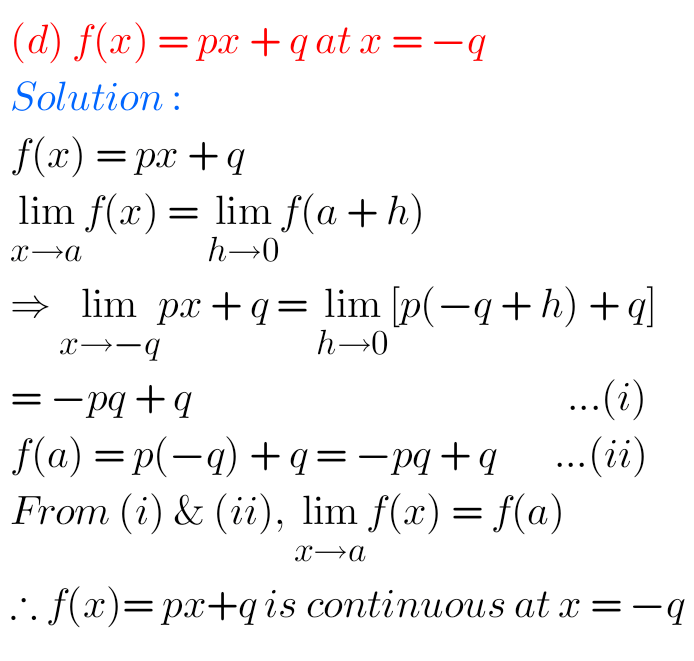## Maths Solutions for Nios check your progress 25.3 Limits and Continuity Senior Secondary

Nios maths senior secondary Limits and Continuity check your progress 25.3 solutions are given. You should study the textbook lesson Limit and continuity very well. You should also practice all example problems and solutions given in the textbook. Observe the given below solutions and try them in your own method. You can see. NIOS maths …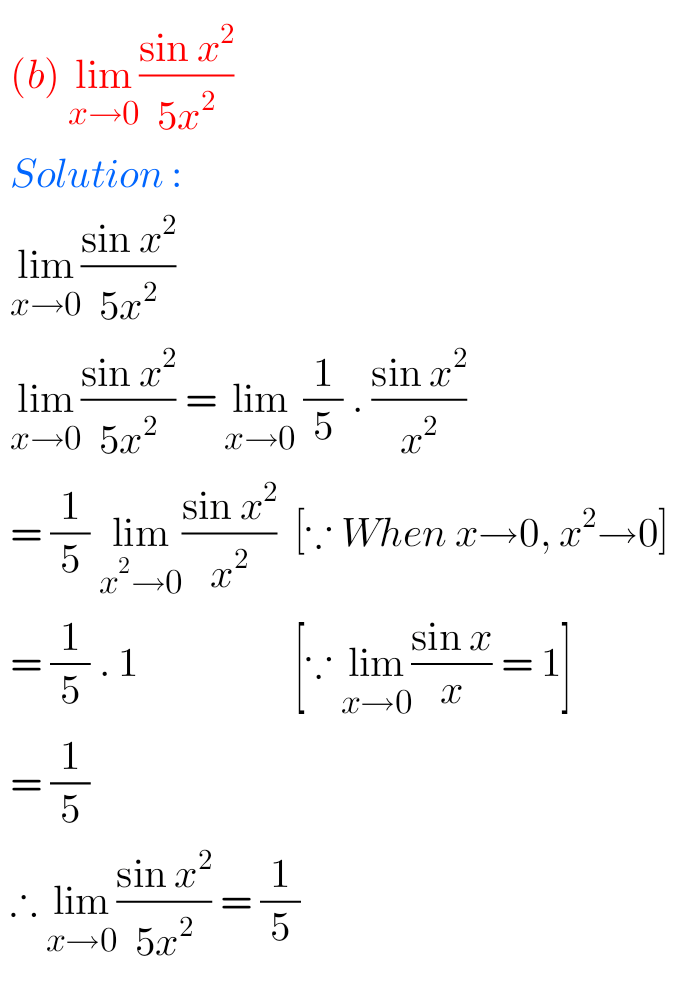## Check Your Progress 25.2 Solutions Limits and Continuity Nios Maths Senior Secondary

NIOS mathematics 311 book 2 chapter 25 Limits and Continuity check your progress 25.2 solutions are given. You should study the textbook lesson Limits and Continuity very well. You should also practice all example problems and solutions given in the textbook. Observe the solutions given below and try them in your own method. NIOS maths …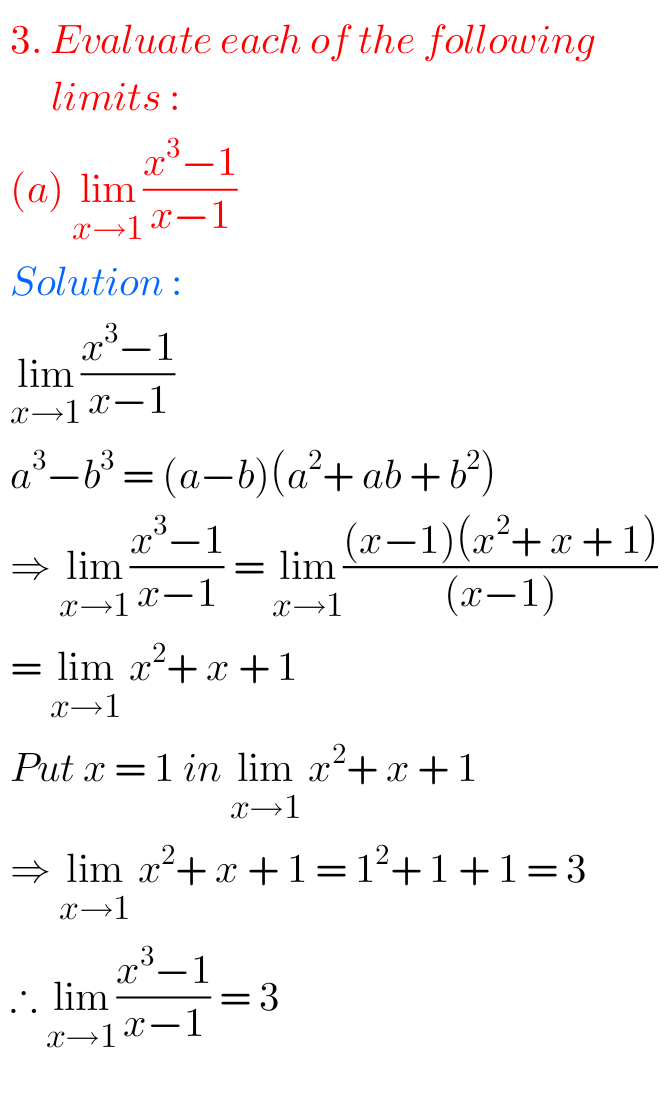## Limits and Continuity NIOS Maths 311 book 2 check your progress 25.1 solutions

NIOS mathematics 311 book 2 chapter 25 Limits and Continuity check your progress 25.1 solutions are given. You should study the textbook lesson Limits and Continuity very well. You should also practice all example problems and solutions given in the textbook. Observe the given below solutions and try them in your own method. You can …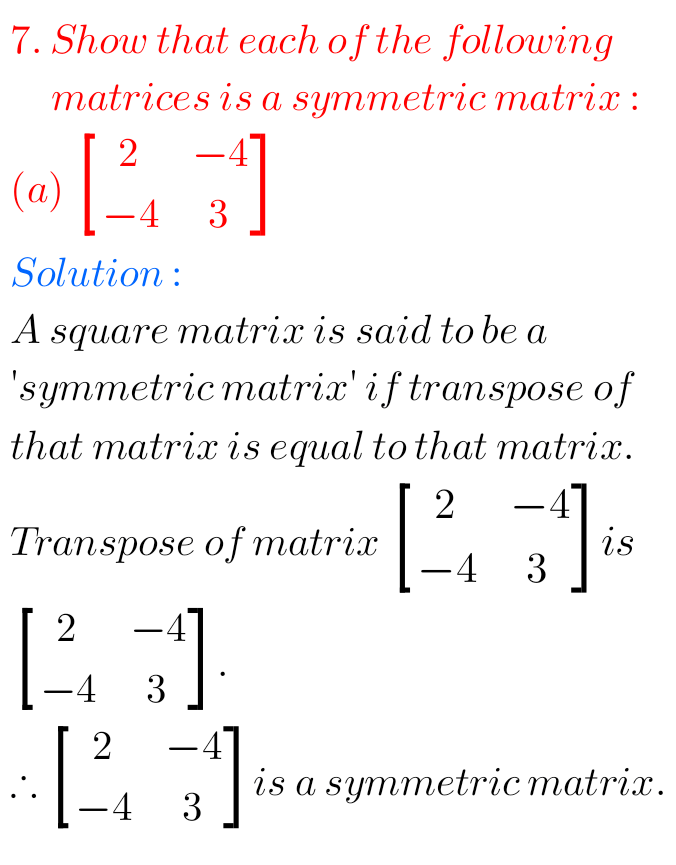## Matrices solutions NIOS check your progress 20.3 book 2 Maths 311

Senior secondary Matrices check your progress 20.3 solutions NIOS Mathematics 311 NIOS book 2 Module VI Algebra II Chapter 20 Matrices check your Progress 20.3 solutions are given. You should study the textbook lesson Matrices very well. You should practice the example problems and solutions given in the textbook. Observe the given solutions and try …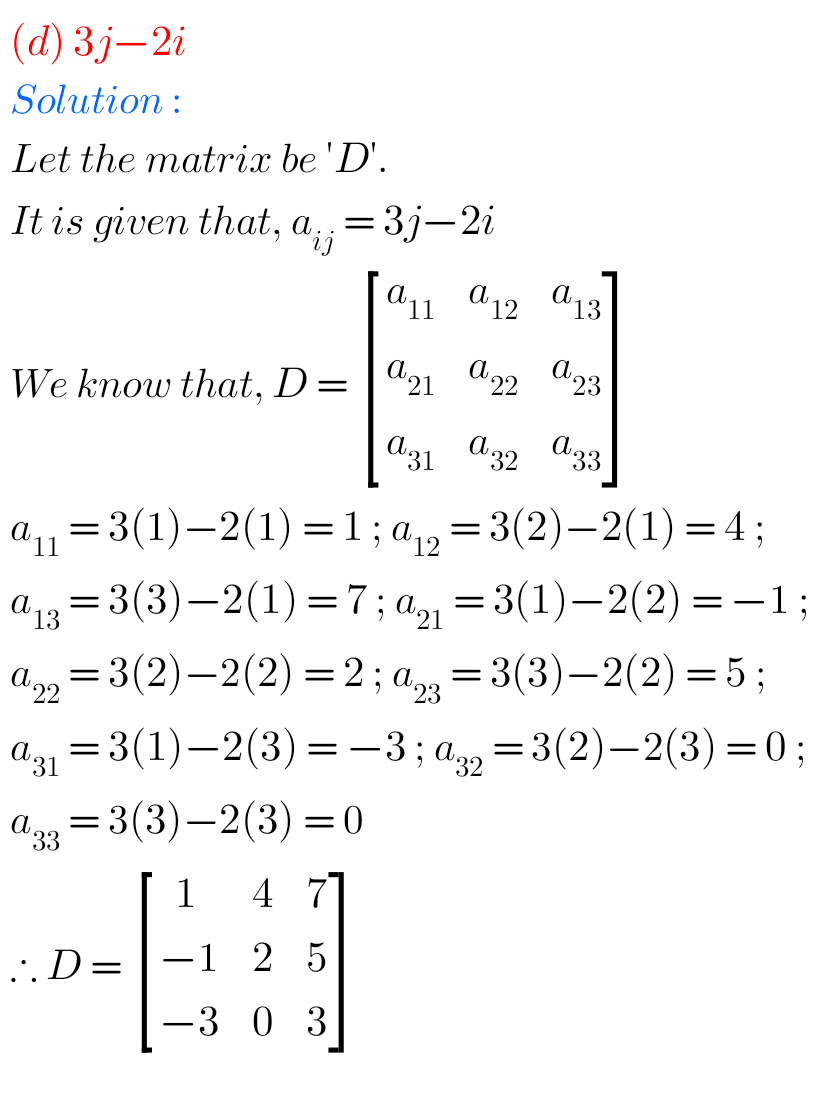## NIOS Maths 311 Book 2 Chapter 20 Matrices Check your process 20.1 solutions

Matrices Check your process 20.1 Solutions senior secondary maths NIOS Mathematics book 2 Module – VI Algebra II Chapter 20 Matrices check your Progress 20.1 solutions are given. You should study the textbook lesson Matrices very well. You should also practice the example problems and solutions given in the textbook. Observe the given solutions and …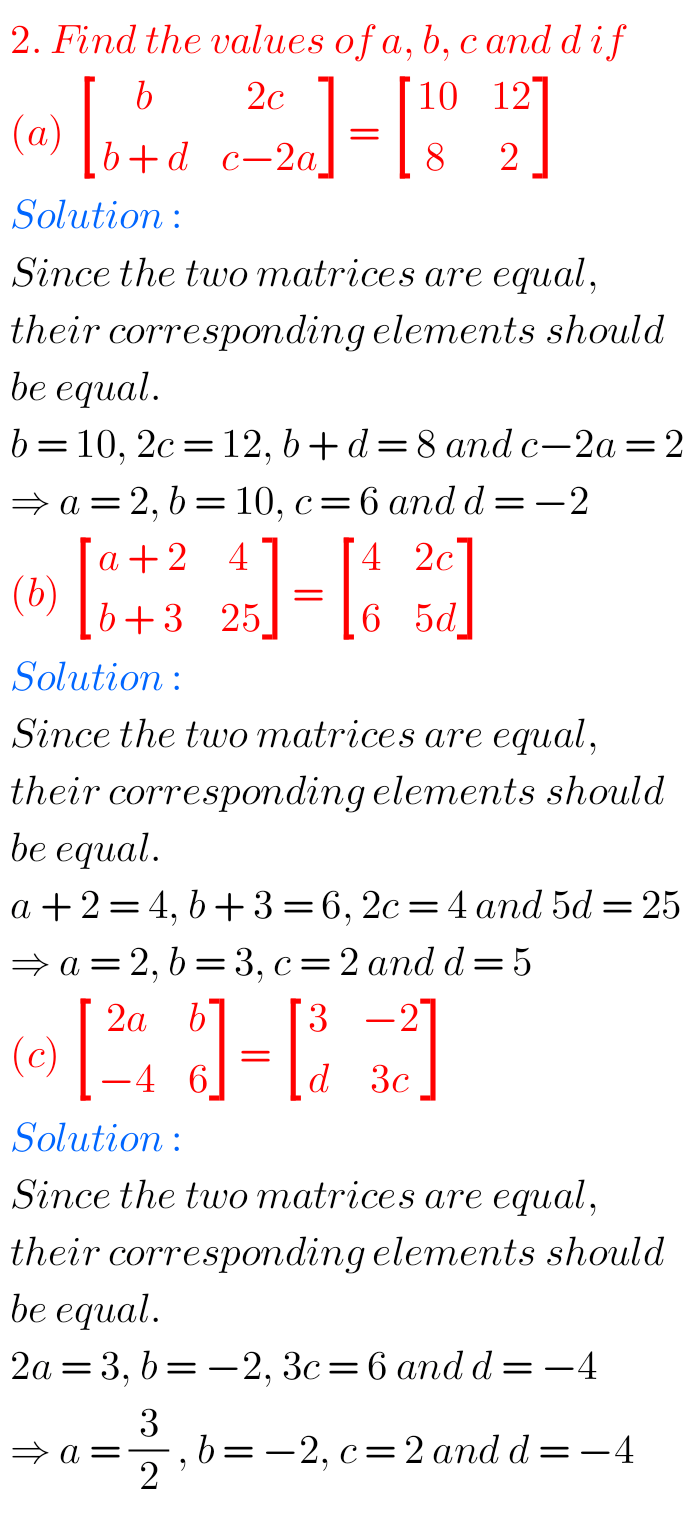## Check your progress 20.2 Matrices solutions Maths 311 book 2 NIOS

Matrices Check your process 20.2 Solutions senior secondary maths 311 NIOS NIOS Mathematics book 2 Module VI Algebra II Chapter 20 Matrices check your Progress 20.2 solutions are given. You Should study the textbook lesson Matrices very well. You should also practice the example problems and solutions given in the textbook. Observe the given solutions …## NIOS Solutions for Terminal Exercise Matrices maths 311 book 2

NIOS senior secondary mathematics 311 book 2 Module – VI Chapter – 20 Matrices Terminal Exercise solutions are given. You should study the textbook lesson Matrices very well. You should also observe and practice all example problems and solutions given in the textbook. Observe the solutions and try them in your own method. You can …